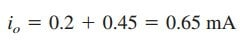# Ideal Op Amp CharacteristicsAfter learning what is operational amplifier, we will learn what is an ideal op amp first.

## Ideal Op Amp

To facilitate the understanding of op amp circuits, we will assume ideal op amps. An op amp is ideal if it has the following characteristics:

1. Infinite open-loop gain, A ≃ ∞.
2. Infinite input resistance, Ri ≃ ∞.
3. Zero output resistance, Ro ≃ 0.

An ideal op amp is an amplifir with infiite open-loop gain, infinite input resistance, and zero output resistance.

Although assuming an ideal op amp provides only an approximate analysis, most modern amplifiers have such large gains and input impedances that the approximate analysis is a good one. Unless stated otherwise, we will assume from now on that every op amp is ideal.

For circuit analysis, the ideal op amp is illustrated in Figure.(1), which is derived from the nonideal model in Figure.(4) in ‘What is Operational Amplifier’.

Two important properties of the ideal op amp are:

1. The currents into both input terminals are zero:

This is due to infinite input resistance. An infinite resistance between the input terminals implies that an open circuit exists there and current cannot enter the op amp. But the output current is not necessarily zero according to Equation.(1) in ‘What is Operational Amplifier’.

2. The voltage across the input terminals is equal to zero; i.e.,

or

Thus, an ideal op amp has zero current into its two input terminals and the voltage between the two input terminals is equal to zero. Equations.(1) and (2) are extremely important and should be regarded as the key handles to analyzing op amp circuits.

## Ideal Op Amp Example

If the same 741 op amp in the ‘What is Operational Example‘ is used in the circuit of Figure.(2) below, calculate the closed-loop gain vo/vs.

Find io when vs = 1 V. Rework it using the ideal op amp model

Solution:

We just
need to keep Equations.(1) and (3) in mind as we analyze the circuit in Figure.(2). Thus, the Figure.(2) the circuit is presented as in Figure.(3).

Notice that

Since i1 = 0, the 40 – and 5 -kΩ resistors are in series; the same current flows through them. v1 is the voltage across the 5 -kΩ resistor. Hence, using the voltage division principle,

According to Equation.(3),